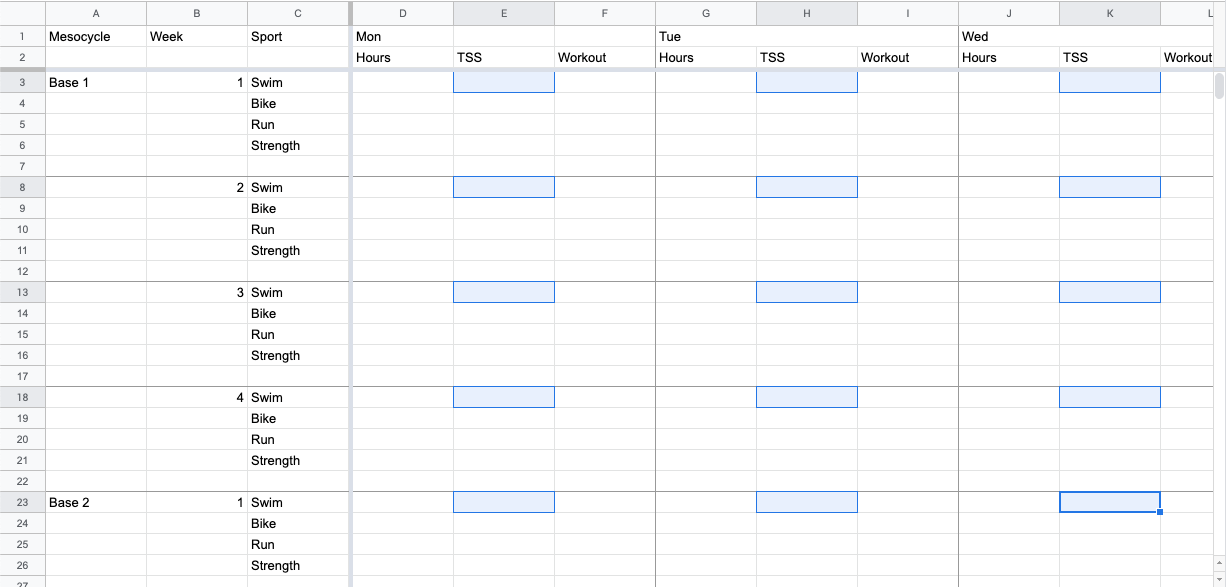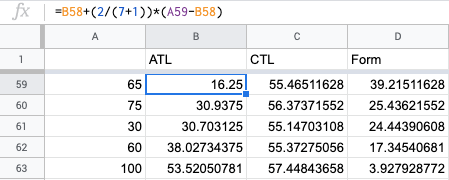# Using a Python Loop to Output a Complex Iterating Formula for a Spreadsheet for Tracking and Calculating Endurance Athlete Training Load

While I mostly want to demonstrate how I hacked a spreadsheet problem with Python, some setup seems necessary to provide context as to why I found that necessary in the first place and the statistics themselves are interesting to analyze from an exercise science perspective. Ergo:

In addition to development, I create training plans for endurance events such as cycling, nordic skiing, and triathlon, the basic component of which follows the principle that the body will adapt to stress over time. One method of calculating this stress, popularized by the TrainingPeaks software and Joel Friel, is Training Stress Score (TSS). Training Stress Score is a product of effort and time. For example, a one hour bike ride at an aerobic pace would have a TSS of 50-60.

In order to see how that stress is affecting the athlete during the course of a training cycle, TrainingPeaks uses a moving average of the stress load. A moving average smoothes short-term fluctuations and highlights the long term trend.

In the TrainingPeaks software, an exponentially weighted moving average of TSS from the last 42 days is used to calculate Chronic Training Load (CTL), or long-term stress, and an exponentially weighted moving average of TSS from the last 7 days is used to calculate Acute Training Load, or short-term stress. Together, the two metrics provide an idea of fitness and fatigue.

With that setup, I have a spreadsheet I use for initially sketching out a training plan macrocycle that looks like this:To calculate the projected Acute Training Load for the Swim workouts I need to gather together cells E3, H3, K3, ..., E8, H8, K8, ..., and so on (highlighted in the above graphic). There's a pattern, but it doesn't iterate in such a way to make filling out a column of values automatic. The path needs to begin with the row for the specific sport and grab data from each column with a TSS value before advancing on to the next row for that sport. The rows are consistently 5 apart. The columns are specific: 'E', 'H', 'K', etc. To collect the data, I wrote a nested loop in Python to output a series of equations that can be pasted into a column. The loop first iterates at the row level, and within each row then iterates over each column in that row before moving on to the next row. In it's simplest form:

``````# first row of data by sport
SWIM_START = 3
BIKE_START = 4
RUN_START = 5

# last row of data on sheet 1
SHT_1_END = 122
# sheet 1 col names with TSS values
COL_NAMES = ['E', 'H', 'K', 'N', 'Q', 'T', 'W']

# every 5th row will be the same sport
ROW_REPEATS_EVERY = 5

# set the first row based on the sport
row = SWIM_START

while row < SHT_1_END:
for col in COL_NAMES:
print(
"=Sheet1!{s1c}{s1r}".format(
s1c=col, s1r=row
)
)
row += ROW_REPEATS_EVERY
``````

Which outputs a list like the following which can then be copy-pasted into a new spreadsheet column:

```=Sheet1!E3
=Sheet1!H3
=Sheet1!K3
=Sheet1!N3
=Sheet1!Q3
=Sheet1!T3
=Sheet1!W3
=Sheet1!E8
=Sheet1!H8
=Sheet1!K8
=Sheet1!N8
=Sheet1!Q8
=Sheet1!T8
=Sheet1!W8
=Sheet1!E13
=Sheet1!H13
=Sheet1!K13
=Sheet1!N13
=Sheet1!Q13
=Sheet1!T13
=Sheet1!W13
=Sheet1!E18
=Sheet1!H18
=Sheet1!K18
=Sheet1!N18
=Sheet1!Q18
=Sheet1!T18
=Sheet1!W18
...
```

From that column, I can then easily create additional columns to calculate ATL or CTL for each sport which can be applied down the column by dragging the cell:```LOADtoday = LOADyesterday + ɑ(STRESStoday - LOADyesterday)
```

Where ɑ is a constant smoothing factor calculated as:

```2 / (N + 1)
```

From there we can also do some interesting things like calculating form, or Training Stress Balance (TSB) which is the chronic load minus the acute load with a positive value generally indicating "fitness" or "freshness" and a negative value indicating fatigue.

We can also calculating the ratio of acute load to chronic load looking for a ratio of 0.8-1.3 with ratios over 1.5 indicating that the athlete might not be adequately recovered to avoid injury and lower ratios indicating that the athlete is either well prepared or an inadequate stress is being applied to create adaptations.

As with all things coaching, it's the individual's response that matters, so these metrics just provide a theoretical model of the load and response.

The complete Python script uses a named argument to specify which sport is being gathered and creates the formulas for the appropriate cells and writes the output to a file. With a bit of bash scripting as demonstrated in the help string, the file can be opened, its contents copied to the Mac clipboard to be pasted into the spreadsheet, and the file removed all in one line:

``````#!/usr/bin/python3

import getopt
import sys

SWIM = 'swim'
BIKE = 'bike'
RUN = 'run'

# first row of data by sport
SWIM_START = 3
BIKE_START = 4
RUN_START = 5

# last row of data on sheet 1
SHT_1_END = 122
# sheet 1 col names with TSS values
COL_NAMES = ['E', 'H', 'K', 'N', 'Q', 'T', 'W']

# every 5th row will be the same sport
ROW_REPEATS_EVERY = 5

def main(argv):
sports = [SWIM, BIKE, RUN]

# run and copy output to clipboard, removing the doc created by the script afterwards
helpstr = 'python3 gather_tss.py -s "swim"; '\
'cat equations.txt | pbcopy; '\
'rm equations.txt'

try:
opts, args = getopt.getopt(argv, "hs:", ["sport="])
except getopt.GetoptError:
print(helpstr)
sys.exit(2)
for opt, arg in opts:
if opt == '-h':
print(helpstr)
sys.exit()
elif opt in ("-s", "--sport"):
sport = arg
if sport not in sports:
print('Sport must be one of {}'.format(", ".join(sports)))
sys.exit(2)

# In sheet 1: Swim starts on row 3, Bike on 4, Run on 5
if sport == SWIM:
row = SWIM_START
elif sport == BIKE:
row = BIKE_START
elif sport == RUN:
row = RUN_START

f = open("equations.txt", "w+")

while row < SHT_1_END:
for col in COL_NAMES:
f.write(
"=Sheet1!{s1c}{s1r}\r\n".format(
s1c=col, s1r=row
)
)
row += ROW_REPEATS_EVERY

f.close()

if __name__ == '__main__':
main(sys.argv[1:])
``````

The script might be further optimized to output the formulas for all 3 sports into respective columns or individual spreadsheet files in one go by iterating over the sports as well. However, it really only needs to be used once (well, thrice really) to build the master spreadsheet. Even passing named arguments might be a bit excessive for a script that will be ran three times. But, as with many things programming, this didn't come together perfectly in the first go and began as something more complex where I was trying to create columns with formulas for the acute training load and chronic training load without a column for TSS in the second sheet as well as compare a simple moving average to exponential moving average. The following is ungainly and inefficient but included for the sake of comparison:

``````#!/usr/bin/python3
import getopt
import sys

SWIM = 'swim'
BIKE = 'bike'
RUN = 'run'

SHORT = 'short'
LONG = 'long'

SIMPLE = 'simple'
EXPONENTIAL = 'exp'

# In sheet 2: The first row with equations (3)
SHT_2_START = 3
# last row of data on sheet 1
SHT_1_END = 119
# last row of data on sheet 2
SHT_2_END = 171
# sheet 1 col names with TSS values
COL_NAMES = ['E', 'H', 'K', 'N', 'Q', 'T', 'W']

SMOOTHING = 2

def main(argv):
sports = [SWIM, BIKE, RUN]
fatigues = [SHORT, LONG]
equations = [SIMPLE, EXPONENTIAL]

# run and copy output to clipboard, removing the doc created by the script afterwards
helpstr = 'python3 print_fatigue_equations.py -s "swim" -f "short" -e "simple"; '\
'cat equations.txt | pbcopy; '\
'rm equations.txt'

try:
opts, args = getopt.getopt(argv, "hs:f:e:", ["sport=", "fatigue=", "equation="])
except getopt.GetoptError:
print(helpstr)
sys.exit(2)
for opt, arg in opts:
if opt == '-h':
print(helpstr)
sys.exit()
elif opt in ("-s", "--sport"):
sport = arg
if sport not in sports:
print('Sport must be one of {}'.format(", ".join(sports)))
sys.exit(2)
elif opt in ("-f", "--fatigue"):
fatigue = arg
if fatigue not in fatigues:
print('Fatigue must be either {}'.format(" or ".join(fatigues)))
sys.exit(2)
elif opt in ("-e", "--equation"):
equation = arg
if equation not in equations:
print('Equation must be either {}'.format(" or ".join(fatigues)))
sys.exit(2)

# In sheet 1: Swim starts on row 3, Bike on 4, Run on 5
# In sheet 2:
#   Swim short term is B, long term is C
#   Bike short term is E, long term is F
#   Run short term is H, long term is I
if sport == SWIM:
sht_1_start = 3
if fatigue == SHORT:
sht_2_col = 'B'
elif fatigue == LONG:
sht_2_col = 'C'
elif sport == BIKE:
sht_1_start = 4
if fatigue == SHORT:
sht_2_col = 'E'
elif fatigue == LONG:
sht_2_col = 'F'
elif sport == RUN:
sht_1_start = 5
if fatigue == SHORT:
sht_2_col = 'H'
elif fatigue == LONG:
sht_2_col = 'I'

if fatigue == SHORT:
days = 5  # TP uses 7
if fatigue == LONG:
days = 40  # TP uses 42

row1 = sht_1_start
row2 = SHT_2_START
ci = 0  # Column Index

f = open("equations.txt", "w+")
while row2 < SHT_2_END:
# simple moving average
if equation == SIMPLE:
f.write(
"={s2c}{s2r}+((Sheet1!{s1c}{s1r}-{s2c}{s2r})/{days})\r\n".format(
s1c=COL_NAMES[ci], s1r=row1, s2c=sht_2_col, s2r=row2, days=days
)
)
elif equation == EXPONENTIAL:
# exponential moving average
f.write(
"=(Sheet1!{s1c}{s1r}*({smoothing}/(1+{days})))+{s2c}{s2r}*(1-({smoothing}/(1+{days})))\r\n".format(
s1c=COL_NAMES[ci], s1r=row1, s2c=sht_2_col, s2r=row2, smoothing=SMOOTHING, days=days
)
)

row2 += 1

if ci < len(COL_NAMES) - 1:
ci += 1
else:
ci = 0

if row1 <= 119:
row1 += 5
else:
row1 = sht_1_start
f.close()

if __name__ == '__main__':
main(sys.argv[1:])
``````

### Feedback?

Email us at enquiries@kinsa.cc.